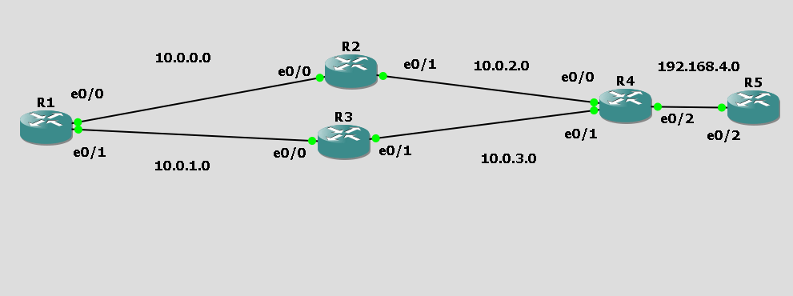# Calculating EIGRP Metric with real world examples

Although not required by the CCNA it’s good to know how to calculate EIGRP metrics to really understand distance. I haven’t found any great guides on teaching this so I thought I’d take a stab at it.

Since the only values we use are K1 and K3 by default (Bw and Delay)

Here is how the calc is done.

BW = 10,000,000/BW

Delay = Delay / 10

(BW + Delay) * 256

Now if we want the Metric over multiple paths we need to remember

BW is always  going to be the lowest across the path

Delay is going to be the sum of all Delays

EXAMPLE TIME!!!From the perspective of R3

I want to calculate my FD and AD of 192.168.4.0

First I see that this whole topology is using Ethernet 10Mbps so the BW is 10,000

The default delay of Ethernet 10Mbps is 1,000.

So let’s calculate the FD to 192.168.4.0 from R4 first…

10,000,000/10,000 =  BW 1,000

1,000/ 10 = D 100

BW 1,000 + D 100 = 1,100 * 256 = FD of R4 to get to 192.168.4.0 281,600

But remember the original question was from the perspective of R3

From R3 Perspective he knows the AD of 192.168.4.0 is 281,600 but of course the router wants the FD.

So the minimum BW across the link is 10,000 (Ethernet 10 Mbps) , we’ve already calculated that as

BW = 1,000. Now we need the SUM of the delays. Earlier our Delay was 1,000/10 = 100 . Now this link has the same delay of 100. So 100 + 100 = 200.

So  let’s do some freaking math man.

1,000 + 200 = 1,200 * 256 = 307,200

So the FD of R3 to get to 192.168.4.0 is 307,200

🙂

EXAMPLE 2 :

Let’s do the FD from R1 now.

Same Ethernet 10 Mbps links.

AD = 307,200 since we already did that math.

1,000 + 300 = 1,300 * 256 = 332,800

OH SO SIMPLE!!!

Mess with the delay if you want to change how paths are taken.